Examples

Chapter 6 Class 8 Squares and Square Roots
Serial order wise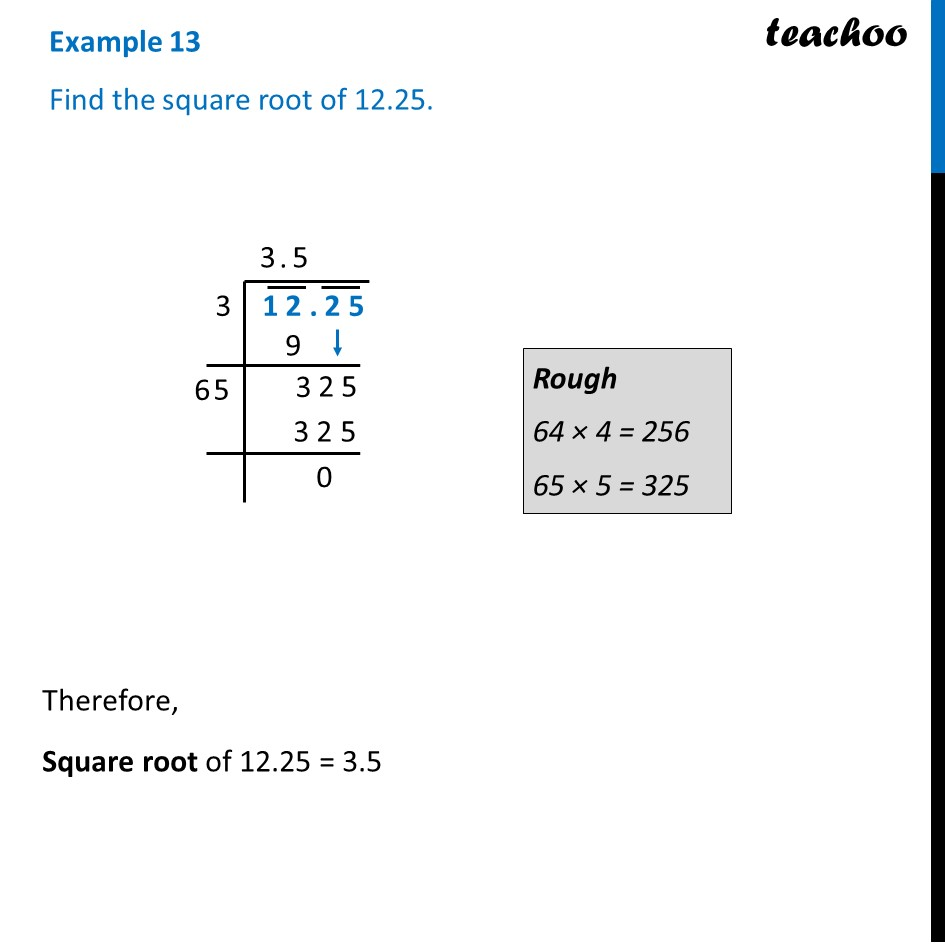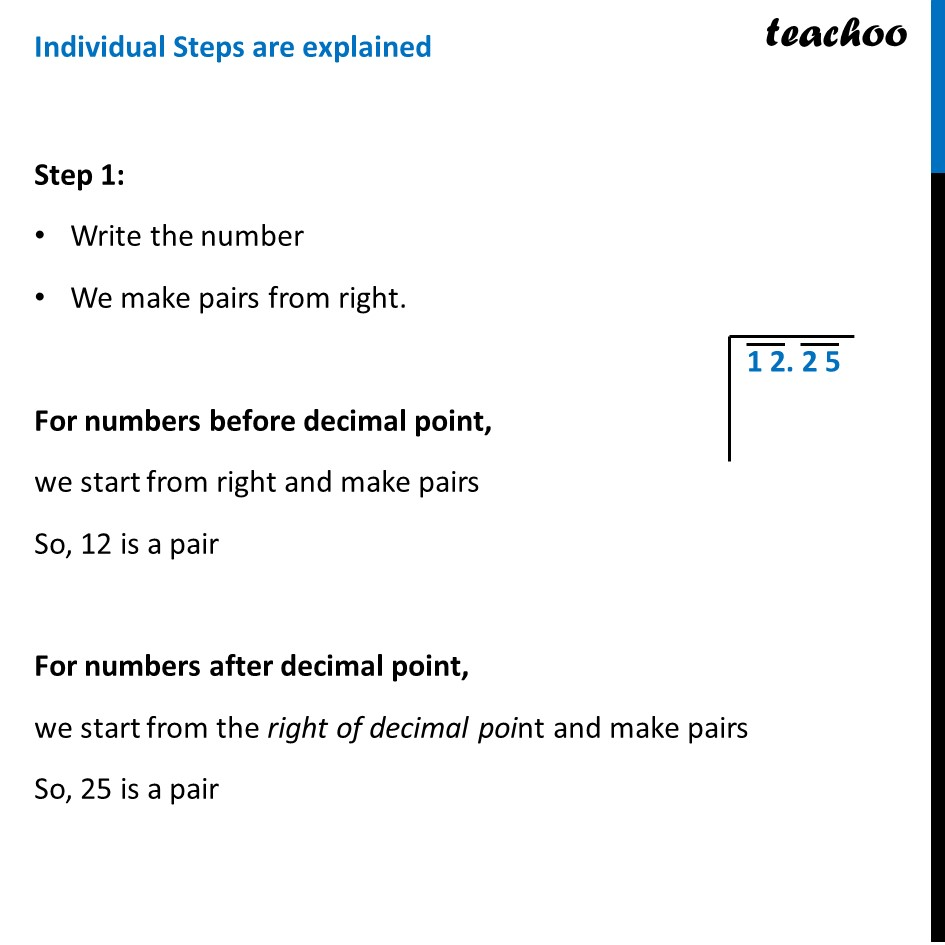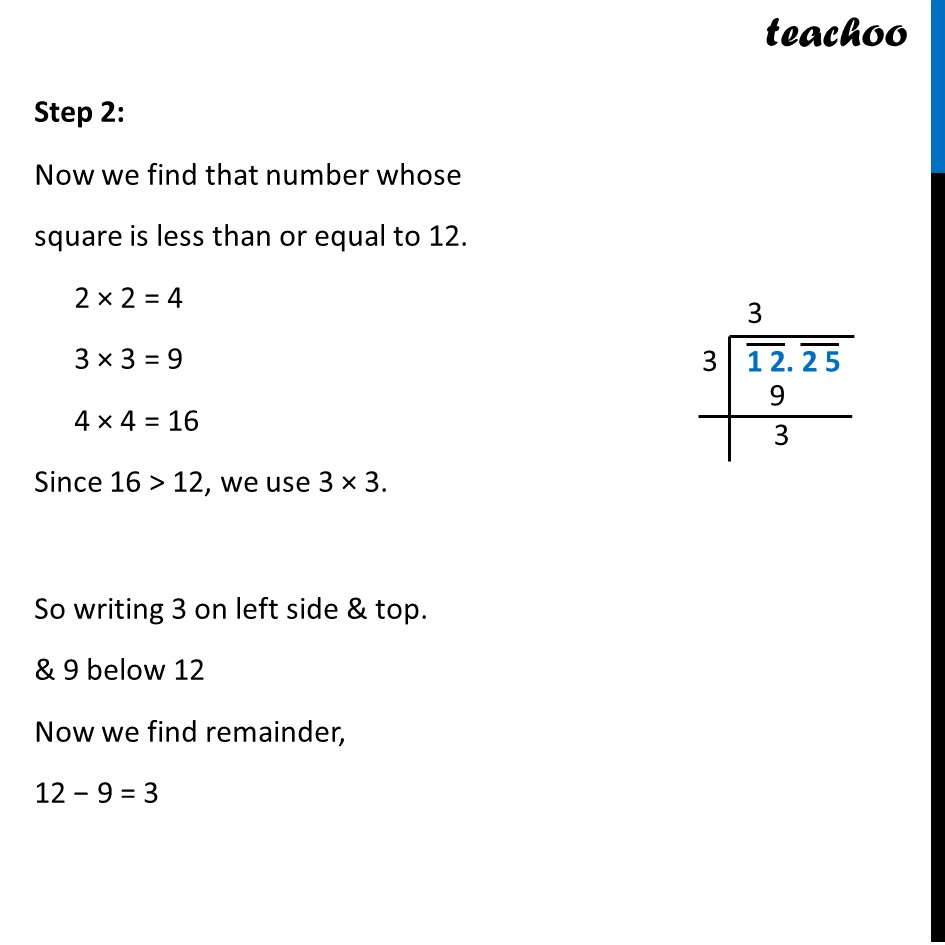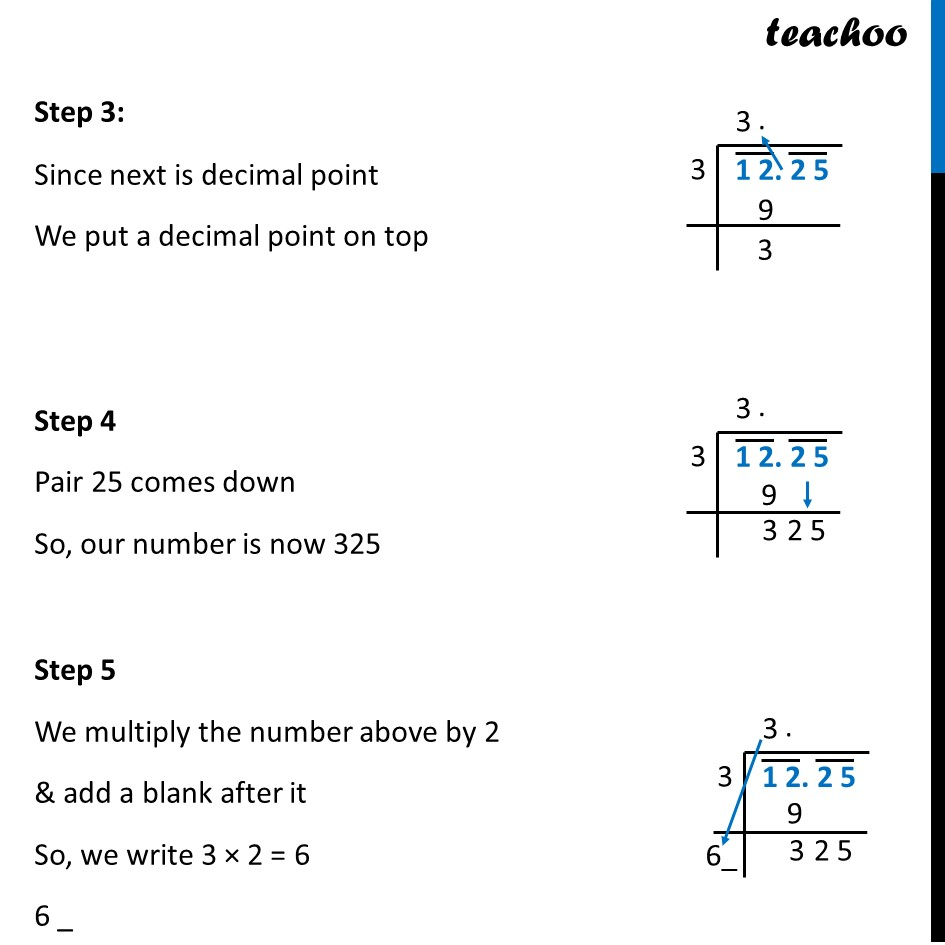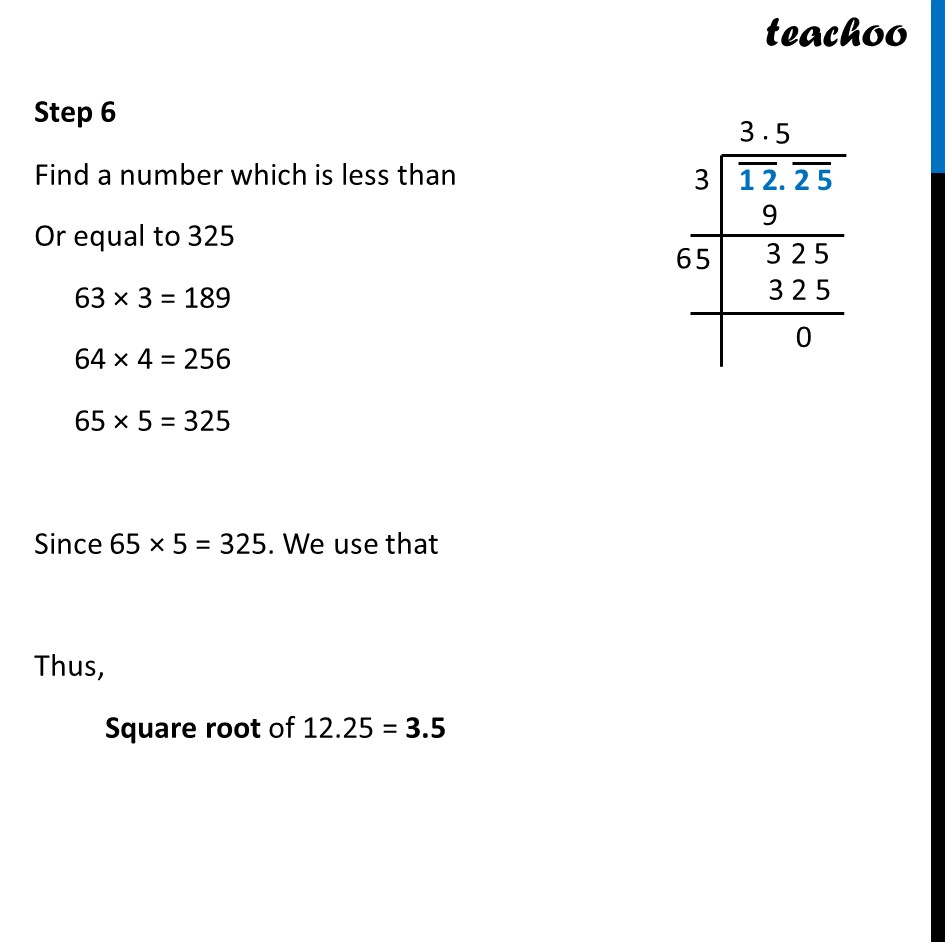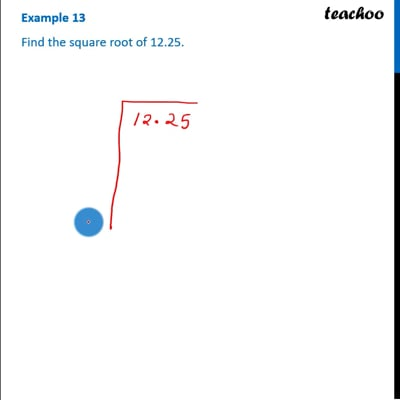This video is only available for Teachoo black users

### Transcript

Example 13 Find the square root of 12.25.Rough 64 × 4 = 256 65 × 5 = 325 Therefore, Square root of 12.25 = 3.5 Individual Steps are explained Step 1: Write the number We make pairs from right. For numbers before decimal point, we start from right and make pairs So, 12 is a pair For numbers after decimal point, we start from the right of decimal point and make pairs So, 25 is a pair Step 2: Now we find that number whose square is less than or equal to 12. 2 × 2 = 4 3 × 3 = 9 4 × 4 = 16 Since 16 > 12, we use 3 × 3. So writing 3 on left side & top. & 9 below 12 Now we find remainder, 12 − 9 = 3 Step 3: Since next is decimal point We put a decimal point on top Step 4 Pair 25 comes down So, our number is now 325 Step 5 We multiply the number above by 2 & add a blank after it So, we write 3 × 2 = 6 6 _ Step 6 Find a number which is less than Or equal to 325 63 × 3 = 189 64 × 4 = 256 65 × 5 = 325 Since 65 × 5 = 325. We use that Thus, Square root of 12.25 = 3.5